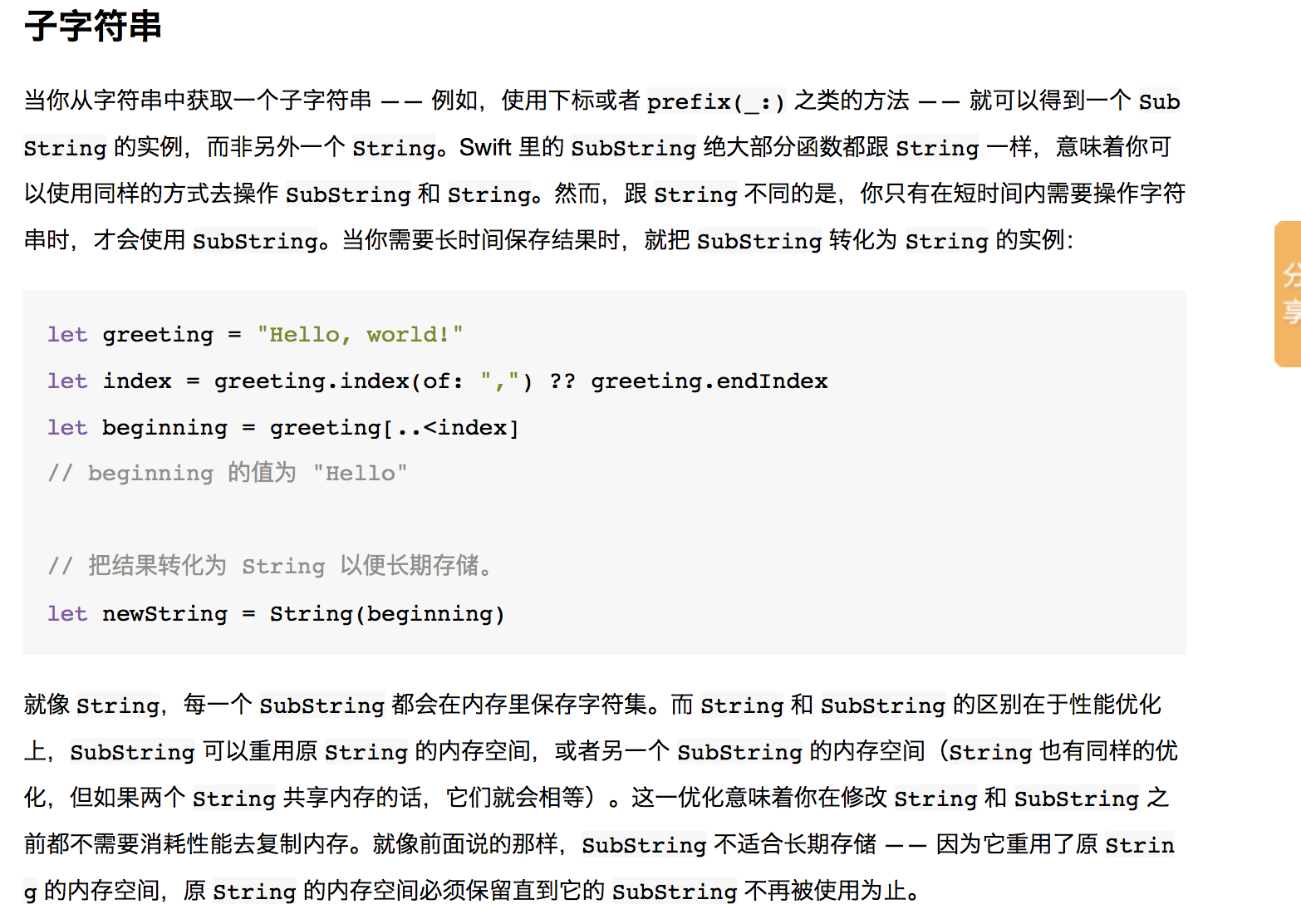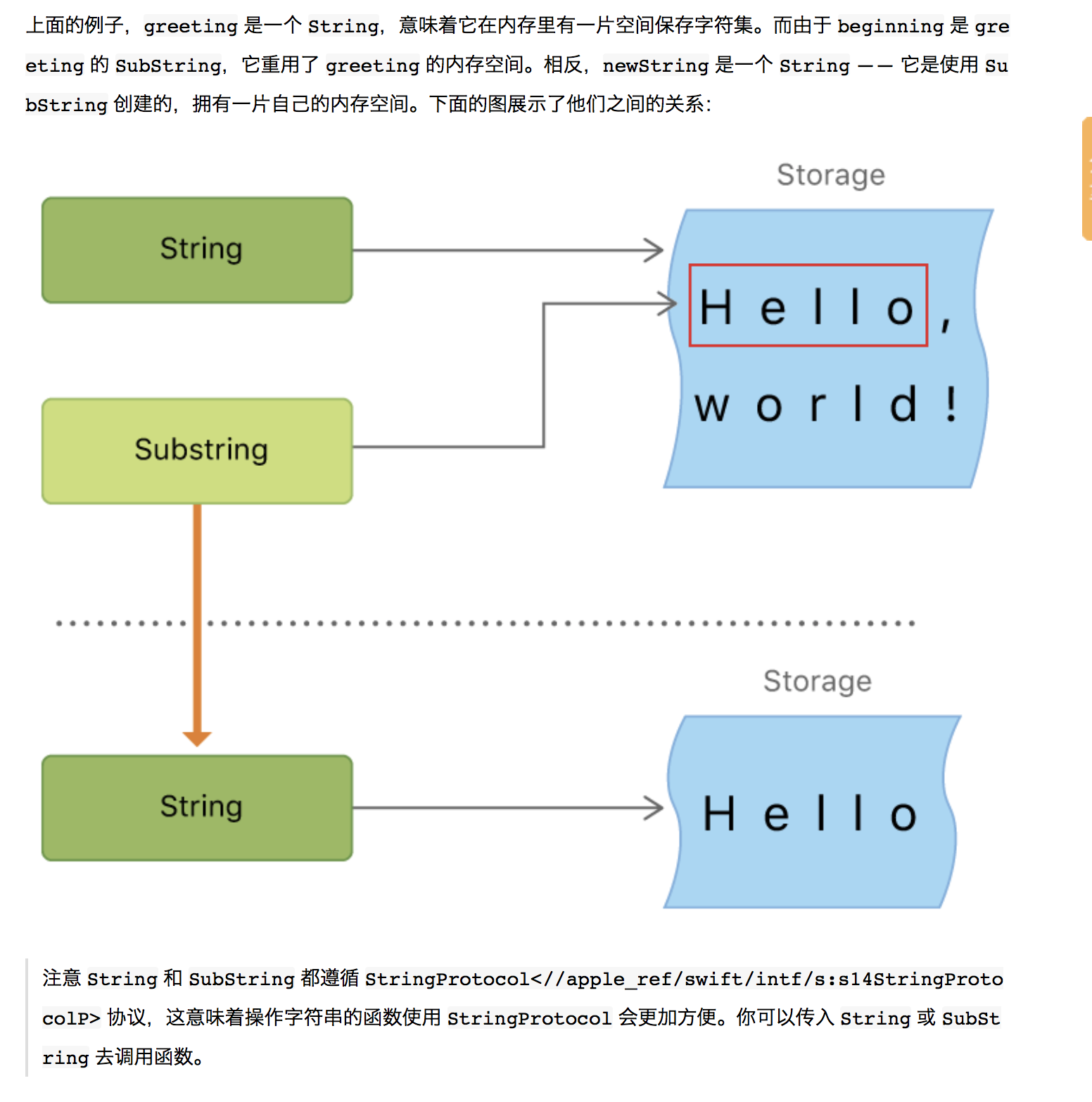2018-03-15 14:22:44 meiyulong518 阅读数 263
• ###### iOS8开发技术（Swift版）:本地化

本讲主要介绍了本地化iOS中各种资源，如字符串、图像、storyboard等

7441 人正在学习 去看看 李宁

# Swift 字符串和子字符swift4 子字符串 相关内容

2019-12-20 14:42:44 u012078168 阅读数 9
• ###### iOS8开发技术（Swift版）:本地化

本讲主要介绍了本地化iOS中各种资源，如字符串、图像、storyboard等

7441 人正在学习 去看看 李宁

Xcode 11.0
Swift 5.1

# 创建

``````// 1. 使用字面量
let aa = "aa"
// 2. 通过String实例化
let bb = String(aa)
``````

``````let cc = ""
let dd = String()
``````

``````// 单行写法
let ee1 = "line1\nline2"

// 多行写法
let ee2 = """
line1
line2
"""
``````

``````let ee3 = """
line1
line2
"""
``````

``````let ee4 = """
line1_1 \
line1_2
"""
// 实际打印出一行 line1_1 line1_2
``````

# 可变字符串

Swift中, 常量不可被修改, 变量可修改.

``````// 可变字符串
var ff = "修改前"
ff = "修改后"
print(ff)    // 打印 修改后
``````

# 字符Character

``````// 创建字符
let aChar:Character = "!"
print(aChar)

// 使用字符数组创建字符串
let chars: [Character] = ["A", "p", "p", "l", "e"]
let str = String(chars)
print(str)

// 遍历字符串, 获取每一个字符的值
for character in "Dog!🐶" {
print(character)
}
// D
// o
// g
// !
// 🐶
``````

# 拼接

``````// 两个字符串直接相加
let gg = "gg"
let hh = "hh"
var ii = gg + hh
print(ii)    // 打印 gghh

// 字符串尾追加字符
let aChar: Character = "!"
ii.append(aChar)
print(ii)    // 打印 gghh!
``````

# 索引/插入/删除

1. 索引
OC中, 字符串的索引是整数, 可以如下操作:

``````// 提取字符串：单个，指定位到末尾，开头至指定位置，指定区间
unichar aChar = [str1 characterAtIndex:5];
NSString *str4 = [str1 substringFromIndex:5];
NSString *str5 = [str1 substringToIndex:5];
NSString *str6 = [str1 substringWithRange:NSMakeRange(2, 4)];
``````

Swift中, 字符串的索引不是整数, 而是一个索引类型String.Index。

``````let str = "Hello, world"
let startIndex = str.startIndex
let endIndex = str.endIndex
print(startIndex)    // Index(_rawBits: 1)
print(endIndex)      // Index(_rawBits: 786433)
``````

``````let str = "Hello, world"
let startIndex = str.startIndex
let endIndex = str.endIndex

// 第二个字符索引
let secondIndex = str.index(after: startIndex)
// 最后一个字符索引
let lastIndex = str.index(before: endIndex)
// 从某一索引往前/后偏移(注意偏移值可正可负)多少位所对应的索引
let customIndex = str.index(startIndex, offsetBy: 5)
// 某一个字符在字符串中的索引
let rIndex = str.firstIndex(of: "r")    // 返回可选类型

// 打印索引对应的字符
print(str[startIndex])          // H
print(str[secondIndex])         // e
print(str[lastIndex])           // d
print(str[customIndex])         // ,
if rIndex != nil {
print(str[rIndex!])         // r
}
``````

``````// 遍历索引值
for index in str.indices {
print("\(str[index])", terminator: "")  // 不换行打印
}
// 打印输出 Hello, world
``````

2. 插入

``````// 插入字符
var welcome = "hello"
welcome.insert("!", at: welcome.endIndex)
// welcome 变量现在等于 "hello!"

// 插入字符串
welcome.insert(contentsOf:" there", at: welcome.index(before: welcome.endIndex))
// welcome 变量现在等于 "hello there!"
``````

3. 删除

``````// 删除字符
welcome.remove(at: welcome.index(before: welcome.endIndex))
// welcome 现在等于 "hello there"

// 删除字符串
let range = welcome.index(welcome.endIndex, offsetBy: -6)..<welcome.endIndex
welcome.removeSubrange(range)
// welcome 现在等于 "hello"
``````

# 截取 (子字符串)

``````let str = "Hello, world"
print(str[str.startIndex])    // H
``````

``````let str = "Hello, world"
let customIndex = str.index(str.endIndex, offsetBy: -5)
let endIndex = str.endIndex
let tempStr = str[customIndex..<endIndex]    // 返回 SubString 类型实例
print(tempStr)    // 打印输出 world

// 把SubString转化为 String 以便长期存储。
let newStr = String(tempStr)
``````

``````// 获取字符串的前5个字符
let tempStr2 = str.prefix(5)
let newStr2 = String(tempStr2)

// 获取字符串的后5个字符
let tempStr3 = str.suffix(5)
let newStr3 = String(tempStr3)
``````

# 插值

``````// 插值
var varA   = 20
let constA = 100
var varC:Float = 20.0

var stringA = "\(varA) 乘于 \(constA) 等于 \(varC * 100)"
print( stringA )
// 20 乘于 100 等于 2000.0
``````

# 字符串比较

``````// 相等
let aa = "Hello"
let bb = "Hello"
if aa == bb {
print("aa等于bb")
}

// 前缀/后缀
let cc: String = "Hello, world"
if cc.hasPrefix("He") {
print("有前缀He")
}
if cc.hasSuffix("ld") {
print("有后缀ld")
}

// 包含字符
if cc.contains("r") {
print("包含字符r")
}
// 包含字符串 (要导入Foundation)
import Foundation
if cc.contains("llo") {
print("包含字符串llo")
}
``````

# 字符串函数

``````isEmpty

hasPrefix(prefix: String)

hasSuffix(suffix: String)

Int(String)

String.count

utf8
UTF-8 编码

utf16
utf16 编码

unicodeScalars
Unicode 标量编码
``````

# 字符串运算符

``````+

+=

==

<

!=

``````

swift4 子字符串 相关内容

2017-02-21 13:05:24 bitcser 阅读数 4425
• ###### iOS8开发技术（Swift版）:本地化

本讲主要介绍了本地化iOS中各种资源，如字符串、图像、storyboard等

7441 人正在学习 去看看 李宁

### Swift3.0语言教程替换子字符串

Swift3.0语言教程替换子字符串,替换子字符串其实就是将字符串中的子字符串删除，然后再进行添加。为了让这一繁琐的过程变的简单，NSString提供了替换子字符串的3个方法，这3个方法分别为：replacingOccurrences(of:with:)、replacingOccurrences(of:with:options:range:)和replacingCharacters(in:with:)方法。

（1）replacingOccurrences(of:with:)方法用来实现子字符串的替换，并返回一个新的字符串，其语法形式如下：

func replacingOccurrences(of target: String, with replacement: String) -> String

【示例1-53】以下将使用replacingOccurrences(of:with:)方法实现子字符串的替换。

import Foundation

var a=NSString(string:"Hello,Tom")

print("替换前：\(a)")

var b=a.replacingOccurrences(of: "Tom", with: "Dave")                                                     //替换

print("替换后：\(b)")

（2）replacingOccurrences(of:with:options:range:)方法和replacingCharacters(in:with:)方法类似，也是用来实现替换的。但是它比replacingCharacters(in:with:)方法多了两个参数：一个是options参数，该参数可以用来指定一个选项标记（这个标记可以作为替换的条件）；另一个参数为searchRang，用来指定一个替换的范围。其语法形式如下：

func replacingOccurrences(of target: String, with replacement: String, options: NSString.CompareOptions = [], range searchRange: NSRange) -> String

【示例1-54】以下将使用replacingOccurrences(of:with:options:range:)方法实现子字符串的替换功能。

import Foundation

var a=NSString(string:"Hello,Tom")

print("替换前：\(a)")

var b=a.replacingOccurrences(of: "TOM", with: "Dave", options: NSString.CompareOptions.caseInsensitive, range: NSMakeRange(0, a.length))

print("替换后：\(b)")

（3）replacingCharacters(in:with:)方法也可以用来将指定范围的子字符串替换掉，其语法形式如下：

func replacingCharacters(in range: NSRange, with replacement: String) -> String

【示例1-55】以下将使用replacingCharacters(in:with:)方法实现替换功能。

import Foundation

var a=NSString(string:"Hello,Tom")

print("替换前：\(a)")

var b=a.replacingCharacters(in: NSMakeRange(0, a.length),with: "This is book")

print("替换后：\(b)")

swift4 子字符串 相关内容

2019-07-15 17:08:37 PanJianlei1990 阅读数 284
• ###### iOS8开发技术（Swift版）:本地化

本讲主要介绍了本地化iOS中各种资源，如字符串、图像、storyboard等

7441 人正在学习 去看看 李宁

Swift 的 String 类型与 Foundation NSString 类进行了无缝桥接。Foundation 还对 String 进行扩展使其可以访问 NSString 类型中定义的方法。这意味着调用那些 NSString 的方法，你无需进行任何类型转换。

# 字符串字面量

``````let something = "Some string literal value"
``````

## 多行字符串字面量

``````let quotation = """
The White Rabbit put on his spectacles.  "Where shall I begin,

"Begin at the beginning," the King said gravely, "and go on
till you come to the end; then stop."
"""
print(quotation)
``````

``````let softWrappedQuotation = """
The White Rabbit put on his spectacles.  "Where shall I begin, \

"Begin at the beginning," the King said gravely, "and go on \
till you come to the end; then stop."
"""
``````

## 字符串字面量的特殊字符

Unicode标量，写成\u{n}(u为小写)，其中n为任意一到八位十六进制数且可用的Unicode位码。

``````let wiseWords = "\"Imagination is more important than knowledge\" - Einstein"
// "Imageination is more important than knowledge" - Enistein
let dollarSign = "\u{24}"             // \$，Unicode 标量 U+0024
let blackHeart = "\u{2665}"           // ♥，Unicode 标量 U+2665
let sparklingHeart = "\u{1F496}"      // ?，Unicode 标量 U+1F496
``````

``````let threeDoubleQuotes = """
Escaping the first quote \"""
Escaping all three quotes \"\"\"
"""
``````

## 扩展字符串分隔符

``````let threeMoreDoubleQuotationMarks = #"""
Here are three more double quotes: """
"""#
``````

# 初始化空字符串

``````var emptyString = ""
var anotherEmptyString = String() // 两个字符串均为空并且等价
``````

``````if emptyString.isEmpty {
print("Nothing to see here")
}
``````

# 字符串可变性

``````var variableString = "Horse"
variableString += " and carriage"
``````

# 使用字符

``````for character in "Dog!" {
print(character)
}
``````

``````let exclamationMark: Character = "!"
``````

``````let catCharacters: [Character] = ["C", "a", "t", "!"]
let catString = String(catCharacters)
``````

# 连接字符串和字符

``````let string1 = "hello"
let string2 = "there"
var welcome = string1 + string2 // welcome 等于 "hello there"
``````

``````var instruction = "look over"
instruction += string2 // instruction 等于 "look over there"
``````

``````welcome.append(exclamationMark) // welcome 等于 "hello there!"
``````

``````let badStart = """
one
two
"""
let end = """
three
"""
// 打印两行
// one
// twothree
let goodStart = """
one
two

"""
print(goodStart + end)
// 打印三行
// one
// two
// three
``````

# 字符串插值

``````let multiplier = 3
let message = "\(multiplier) times 2.5 is \(Double(multiplier) * 2.5)"
``````

# 计算字符数量

``````let unusualMenagerie = "Koala ?, Snailm?, Penguin ?, Dromedary ?"
print(unusualMenagerie.count)
``````

# 访问和修改字符串

## 字符串索引

``````let greeting = "Guten tag!"
print(greeting[greeting.startIndex])
print(greeting[greeting.index(before: greeting.endIndex)])
print(greeting[greeting.index(after: greeting.startIndex)])
print(greeting[greeting.index(greeting.startIndex, offsetBy: 7)])
print(greeting.endIndex)
``````

``````for index in greeting.indices {
print("\(greeting[index]) ", terminator:"")
}
``````

## 插入和删除

``````var welcome_ = "hello"
welcome_.insert("!", at: welcome_.endIndex)
print(welcome_)
welcome_.insert(contentsOf: " there", at: welcome_.index(before: welcome_.endIndex))
print(welcome_)
``````

``````welcome_.remove(at: welcome_.index(before: welcome_.endIndex))
print(welcome_)
let range = welcome_.index(welcome_.endIndex, offsetBy: -6)..<welcome_.endIndex
welcome_.removeSubrange(range)
print(welcome_)
``````

# 子字符串

``````let greeting_ = "Hello, world!"
let index = greeting_.firstIndex(of: ",") ?? greeting_.endIndex
let beginning = greeting_[..<index]
let newString = String(beginning)
``````

String 和 SubString 都遵循 StringProtocol<//apple_ref/swift/intf/s:s14StringProtocolP> 协议，这意味着操作字符串的函数使用 StringProtocol 会更加方便。你可以传入 String 或 SubString 去调用函数。

# 比较字符串

Swift 提供了三种方式来比较文本值：字符串/字符相等、前缀相等、后缀相等。

## 字符串/字符相等

``````let quotation_ = "we're a lot alike, you and I."
let sameQuotation_ = "we're a lot alike, you and I."
if quotation_ == sameQuotation_ {
print("These two strings are considered equal")
}
``````

## 前缀/后缀相等

``````print(quotation_.hasPrefix("we"), quotation_.hasSuffix("and I."))
``````

hasPrefix(? 和 hasSuffix(? 方法都是在每个字符串中逐字符比较其可扩展的字符群集是否标准相等。

swift4 子字符串 相关内容

2016-04-15 12:18:14 cracj 阅读数 492
• ###### iOS8开发技术（Swift版）:本地化

本讲主要介绍了本地化iOS中各种资源，如字符串、图像、storyboard等

7441 人正在学习 去看看 李宁

let str = "0123456��画画…………"

let s1 = (str as NSString).substringFromIndex(3)

print(s1)

debugPrint(index.dynamicType)

let s2 = str.substringFromIndex(index)

print(s2)

let tart = str.startIndex

let s3 = str.substringWithRange(tart..<end)

print(s3)

swift4 子字符串 相关内容